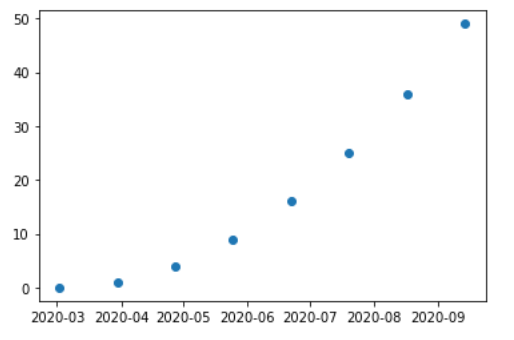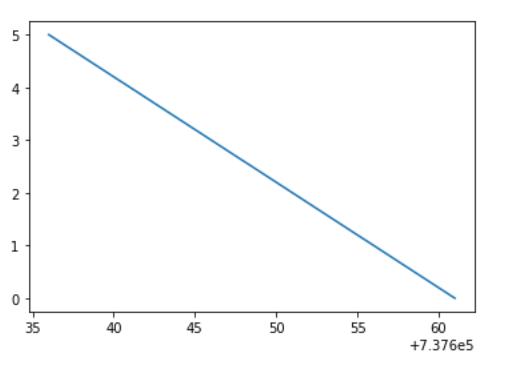Related Articles
Matplotlib.dates.drange() in Python
• Last Updated : 19 Apr, 2020

Matplotlib is an amazing visualization library in Python for 2D plots of arrays. Matplotlib is a multi-platform data visualization library built on NumPy arrays and designed to work with the broader SciPy stack.

## matplotlib.dates.drange()

The `matplotlib.dates.drange()` function returns a sequence of equally spaced Matplotlib dates. The date range starts from ‘dstart’ and go up to, but not including ‘dend’. The space between each date is called delta.

Syntax: matplotlib.dates.drange(dstart, dend, delta)

Parameters:

1. dstart: Starting point of the date range and is a python’s datetime date.
2. dend: Ending point of the date range and is a python’s datetime date.
3. delta: It represents the spacing between each dates and belongs to python’s datetime.timedelta.

Returns: It returns a numpy array which is a list of floats representing Matplotlib dates.

Example 1:

 `import` `datetime``import` `matplotlib.pyplot as plt``from` `matplotlib.dates ``import` `DayLocator, HourLocator, DateFormatter, drange``import` `numpy as np`` ` ` ` `date_1 ``=` `datetime.datetime( ``2020``, ``3``, ``2``)``date_2 ``=` `datetime.datetime( ``2020``, ``10``, ``10``)`` ` `time_delta ``=` `datetime.timedelta(days ``=` `28``)``dates ``=` `drange(date_1, date_2, time_delta)`` ` `y_axis ``=` `np.arange( ``len``(dates) )`` ` `fig, ax ``=` `plt.subplots()``ax.plot_date(dates, y_axis ``*` `y_axis)`` ` `ax.xaxis.set_major_formatter( DateFormatter(``'% Y-% m'``) )`` ` `plt.show()`

Output:Example 2:

 `import` `datetime``import` `matplotlib.pyplot as plt``import` `matplotlib.dates as mdates`` ` `date ``=` `[datetime.datetime(``2020``, ``8``, ``24``, ``0``, ``0``),``        ``datetime.datetime(``2020``, ``8``, ``23``, ``0``, ``0``), ``        ``datetime.datetime(``2020``, ``8``, ``22``, ``0``, ``0``), ``        ``datetime.datetime(``2020``, ``8``, ``21``, ``0``, ``0``), ``        ``datetime.datetime(``2020``, ``8``, ``18``, ``0``, ``0``),``        ``datetime.datetime(``2020``, ``8``, ``17``, ``0``, ``0``),``        ``datetime.datetime(``2020``, ``8``, ``16``, ``0``, ``0``),``        ``datetime.datetime(``2020``, ``8``, ``15``, ``0``, ``0``),``        ``datetime.datetime(``2020``, ``8``, ``14``, ``0``, ``0``),``        ``datetime.datetime(``2020``, ``8``, ``11``, ``0``, ``0``),``        ``datetime.datetime(``2020``, ``8``, ``10``, ``0``, ``0``), ``        ``datetime.datetime(``2020``, ``8``, ``9``, ``0``, ``0``),``        ``datetime.datetime(``2020``, ``8``, ``8``, ``0``, ``0``),``        ``datetime.datetime(``2020``, ``8``, ``7``, ``0``, ``0``),``        ``datetime.datetime(``2020``, ``8``, ``4``, ``0``, ``0``),``        ``datetime.datetime(``2020``, ``8``, ``3``, ``0``, ``0``),``        ``datetime.datetime(``2020``, ``8``, ``2``, ``0``, ``0``),``        ``datetime.datetime(``2020``, ``8``, ``1``, ``0``, ``0``)]`` ` `# is a datetime.datetime object ``# according to type``start_date ``=` `date[``0``]`` ` `# is a datetime.datetime object according ``# to type    ``end_date ``=` `date[``-``1``]``delta ``=` `datetime.timedelta(days ``=` `5``)`` ` `# the drange function``dates ``=` `mdates.drange(start_date, end_date, ``-``delta)``y_data ``=` `range``(``len``(dates))`` ` `plt.plot(dates, y_data)`

Output:Attention geek! Strengthen your foundations with the Python Programming Foundation Course and learn the basics.

To begin with, your interview preparations Enhance your Data Structures concepts with the Python DS Course.

My Personal Notes arrow_drop_up